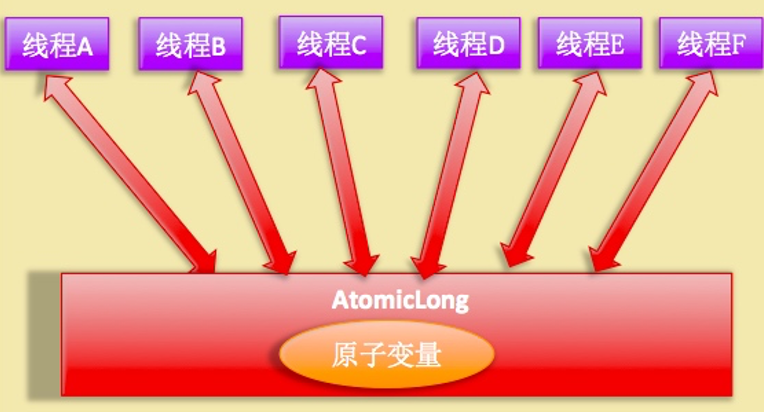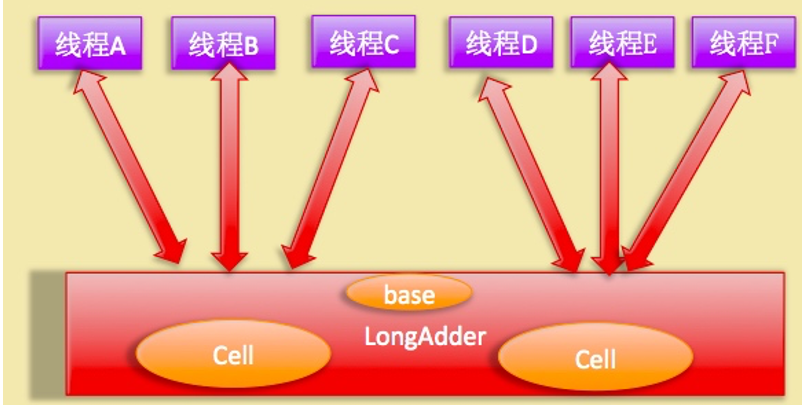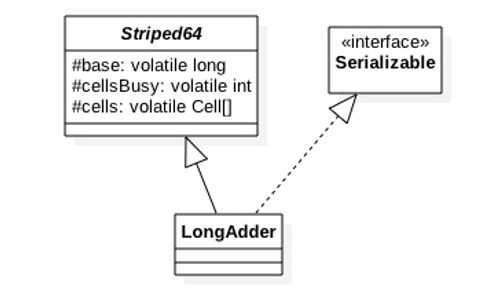public final long incrementAndGet() {
for (;;) {
long current = get();
long next = current + 1;
if (compareAndSet(current, next))
return next;
}
}Cell 类型是Atomic的一个改进，用来减少缓存的争用，对于大多数原子操作字节填充是浪费的，因为原子操作都是无规律的分散在内存中进行的，多个原子性操作彼此之间是没有接触的，但是原子性数组元素彼此相邻存放将能经常共享缓存行，也就是伪共享。所以这在性能上是一个提升。@sun.misc.Contended static final class Cell {
volatile long value;
Cell(long x) { value = x; }
final boolean cas(long cmp, long val) {
return UNSAFE.compareAndSwapLong(this, valueOffset, cmp, val);
}

// Unsafe 技术
private static final sun.misc.Unsafe UNSAFE;
private static final long valueOffset;
static {
try {
UNSAFE = sun.misc.Unsafe.getUnsafe();
Class<?> ak = Cell.class;
valueOffset = UNSAFE.objectFieldOffset
(ak.getDeclaredField("value"));
} catch (Exception e) {
throw new Error(e);
}
}
}

1.long sum() 方法：返回当前的值，内部操作是累加所有 Cell 内部的 value 的值后累加 base，如下代码，由于计算总和时候没有对 Cell 数组进行加锁，所以在累加过程中可能有其它线程对 Cell 中的值进行了修改，也有可能数组进行了扩容，所以 sum 返回的值并不是非常精确的，

源码如下：

public long sum() {
Cell[] as = cells; Cell a;
long sum = base;
if (as != null) {
for (int i = 0; i < as.length; ++i) {
if ((a = as[i]) != null)
sum += a.value;
}
}
return sum;
}

2.void reset() 方法：重置操作，如下代码把 base 置为 0，如果 Cell 数组有元素，则元素值重置为 0。源码如下：

　　public void reset() {
Cell[] as = cells; Cell a;
base = 0L;
if (as != null) {
for (int i = 0; i < as.length; ++i) {
if ((a = as[i]) != null)
a.value = 0L;
}
}
}

3.long sumThenReset() 方法：是sum 的改造版本，如下代码，在计算 sum 累加对应的 cell 值后，把当前 cell 的值重置为 0，base 重置为 0。 当多线程调用该方法时候会有问题，比如考虑第一个调用线程会清空 Cell 的值，后一个线程调用时候累加时候累加的都是 0 值。

源码如下：

　　public long sumThenReset() {
Cell[] as = cells; Cell a;
long sum = base;
base = 0L;
if (as != null) {
for (int i = 0; i < as.length; ++i) {
if ((a = as[i]) != null) {
sum += a.value;
a.value = 0L;
}
}
}
return sum;
}

4.long longValue() 等价于 sum()，源码如下：

    public long longValue() {
return this.sum();
}

5.void add(long x） 累加增量 x 到原子变量，这个过程是原子性的。源码如下：

　　public void add(long x) {
Cell[] as; long b, v; int m; Cell a;
if ((as = cells) != null || !casBase(b = base, b + x)) {//(1)
boolean uncontended = true;
if (as == null || (m = as.length - 1) < 0 ||//(2)
(a = as[getProbe() & m]) == null ||//(3)
!(uncontended = a.cas(v = a.value, v + x)))//(4)
longAccumulate(x, null, uncontended);//(5)
}
}

final boolean casBase(long cmp, long val) {
return UNSAFE.compareAndSwapLong(this, BASE, cmp, val);
}

## 二.LongAccumulator类源码分析

public LongAccumulator(LongBinaryOperator accumulatorFunction,long identity) {
this.function = accumulatorFunction;
base = this.identity = identity;
}
public interface LongBinaryOperator {

//根据两个参数计算返回一个值
long applyAsLong(long left, long right);
}

　　 LongAdder adder = new LongAdder();
LongAccumulator accumulator = new LongAccumulator(new LongBinaryOperator() {

@Override
public long applyAsLong(long left, long right) {
return left + right;
}
}, 0);

　　public void add(long x) {
Cell[] as; long b, v; int m; Cell a;
if ((as = cells) != null || !casBase(b = base, b + x)) {
boolean uncontended = true;
if (as == null || (m = as.length - 1) < 0 ||
(a = as[getProbe() & m]) == null ||
!(uncontended = a.cas(v = a.value, v + x)))
longAccumulate(x, null, uncontended);
}
}

LongAccumulator的accumulate方法的源码如下：

　　public void accumulate(long x) {
Cell[] as; long b, v, r; int m; Cell a;
if ((as = cells) != null ||
(r = function.applyAsLong(b = base, x)) != b && !casBase(b, r)) {
boolean uncontended = true;
if (as == null || (m = as.length - 1) < 0 ||
(a = as[getProbe() & m]) == null ||
!(uncontended =
(r = function.applyAsLong(v = a.value, x)) == v ||
a.cas(v, r)))
longAccumulate(x, function, uncontended);
}
}

else if (casBase(v = base, ((fn == null) ? v + x :fn.applyAsLong(v, x))))
// Fall back on using base　　　　　　break;


posted @ 2018-06-07 19:54  妮蔻  阅读(3327)  评论(1编辑  收藏